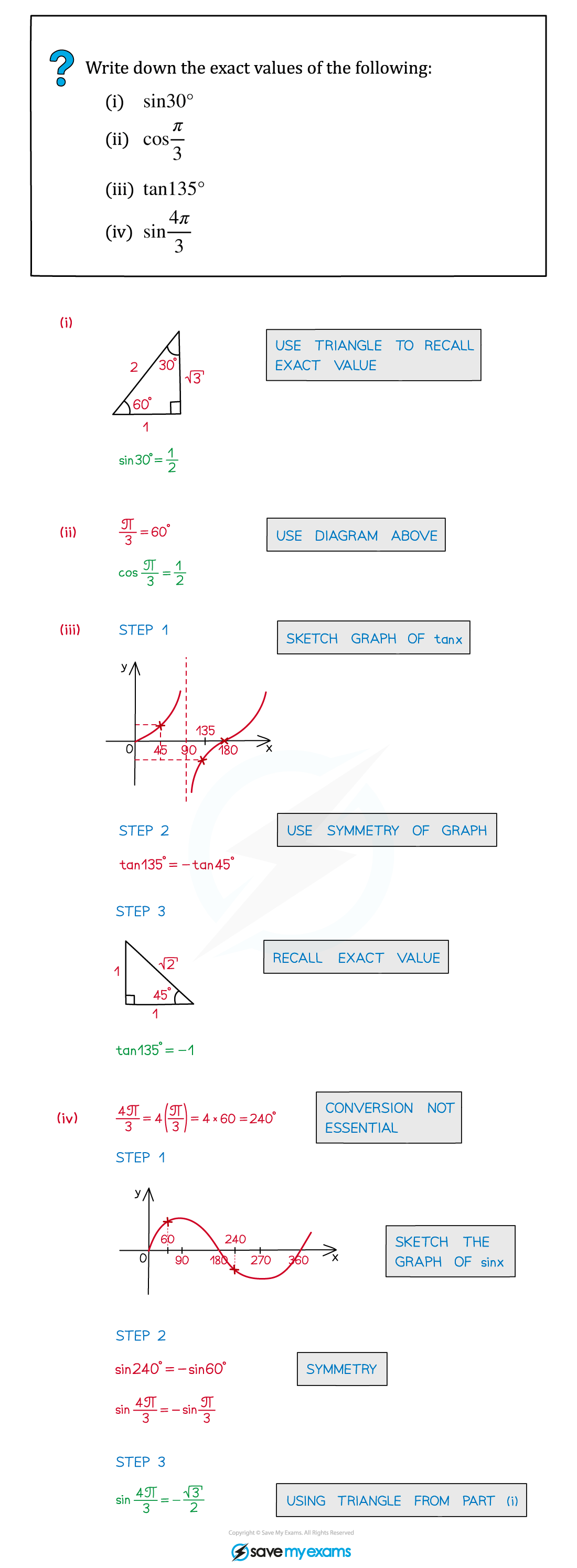# AQA A Level Maths: Pure复习笔记5.4.2 Trigonometry Exact Values

### Trigonometry Exact Values

#### Exact values

• In trigonometry some values of sin, cos and tan are “nice”
• For example sin 30° = ½, tan 45° = 1
• We can find those associated with the angles 30°, 45° and 60° using SOHCAHTOA on isosceles and equilateral triangles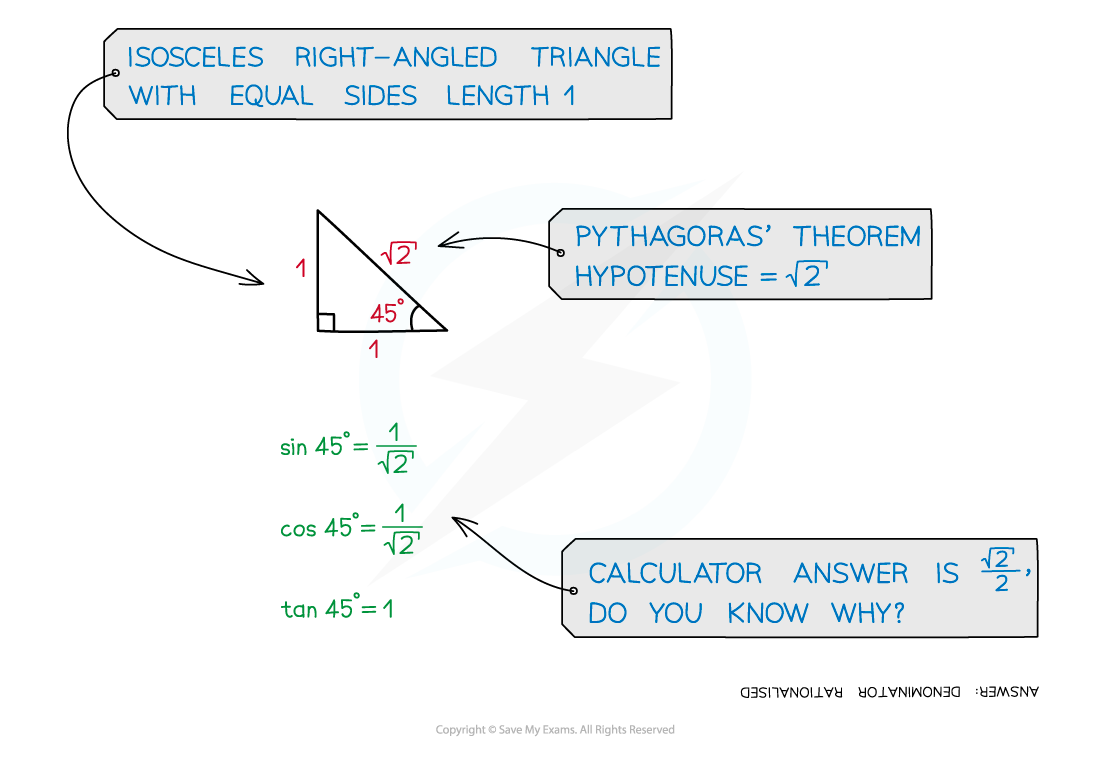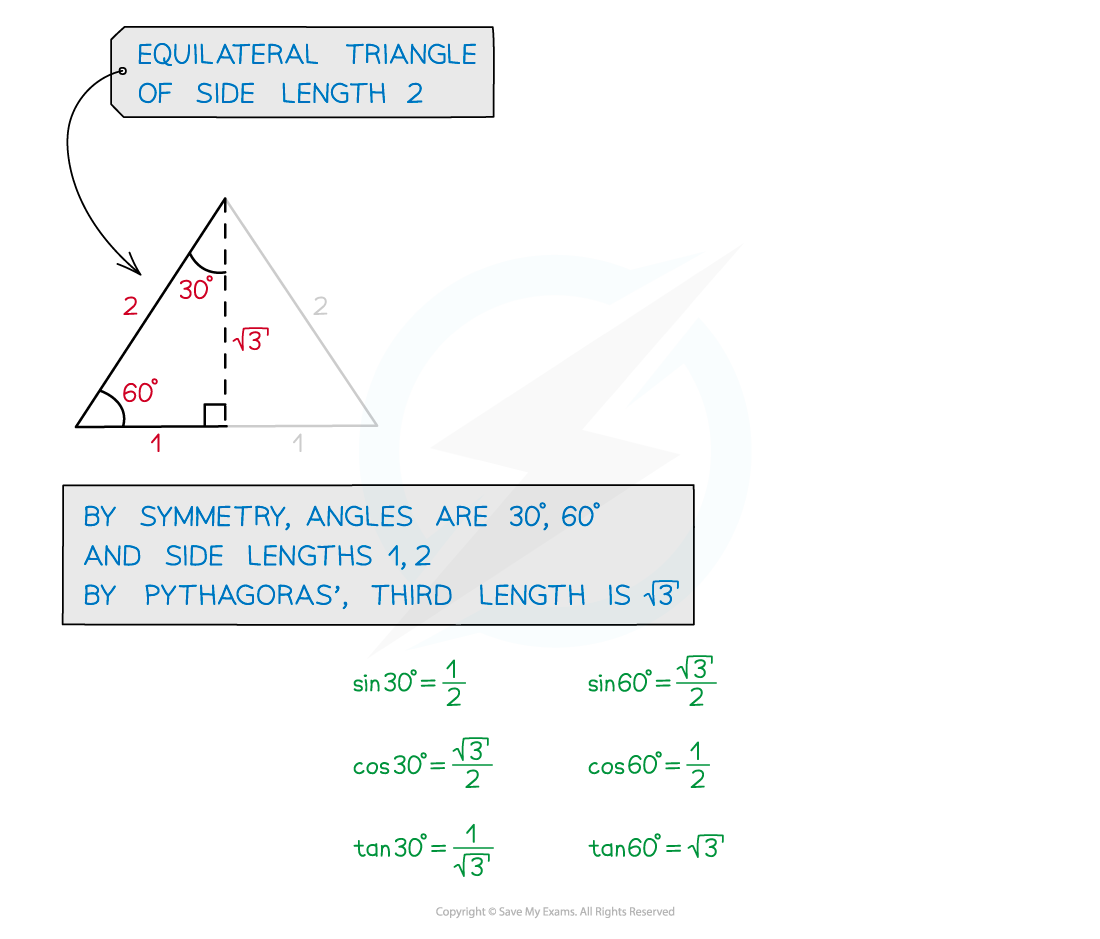• sin, cos and tan values for 30°, 45° and 60° should be recalled easily
• Memorise either the actual values or how to work them out from the triangle
• sin, cos and tan for 0°, 90°, 180° should also be very familiar
• They can be recalled using the relevant trigonometric graph (see Graphs of Trigonometric Functions)

#### Exact values and radians

• All of the above applies to radians as well as degrees
• Here is a table of all exact values including radians (see Radian Measure)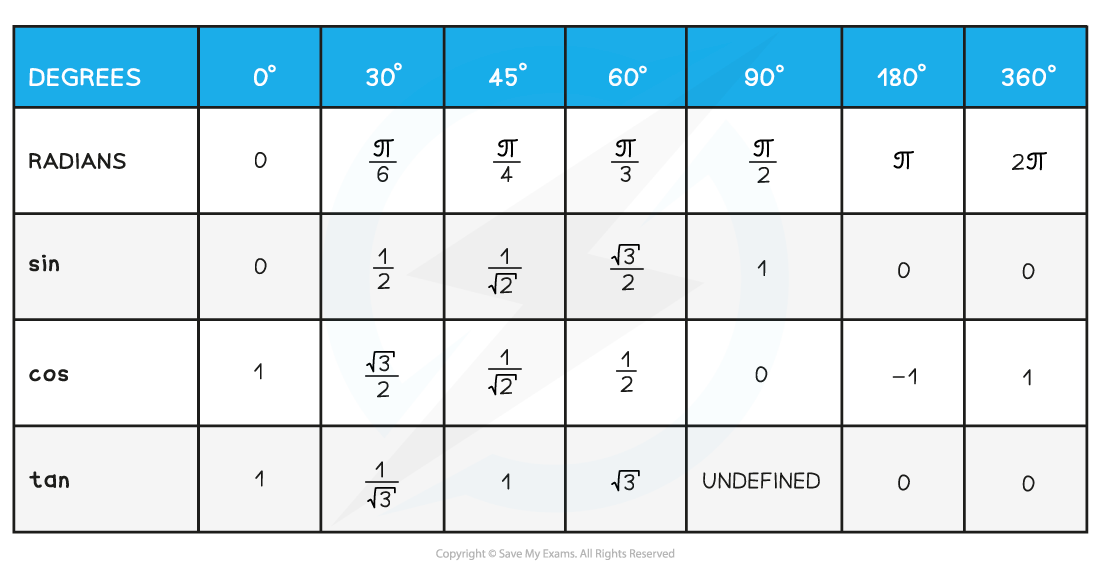#### How do I find exact values of other angles?

• Exact sin, cos and tan values of multiples of 30°, 45°, 60° can also be found
• This is a combination of recalling the basic values and using the graph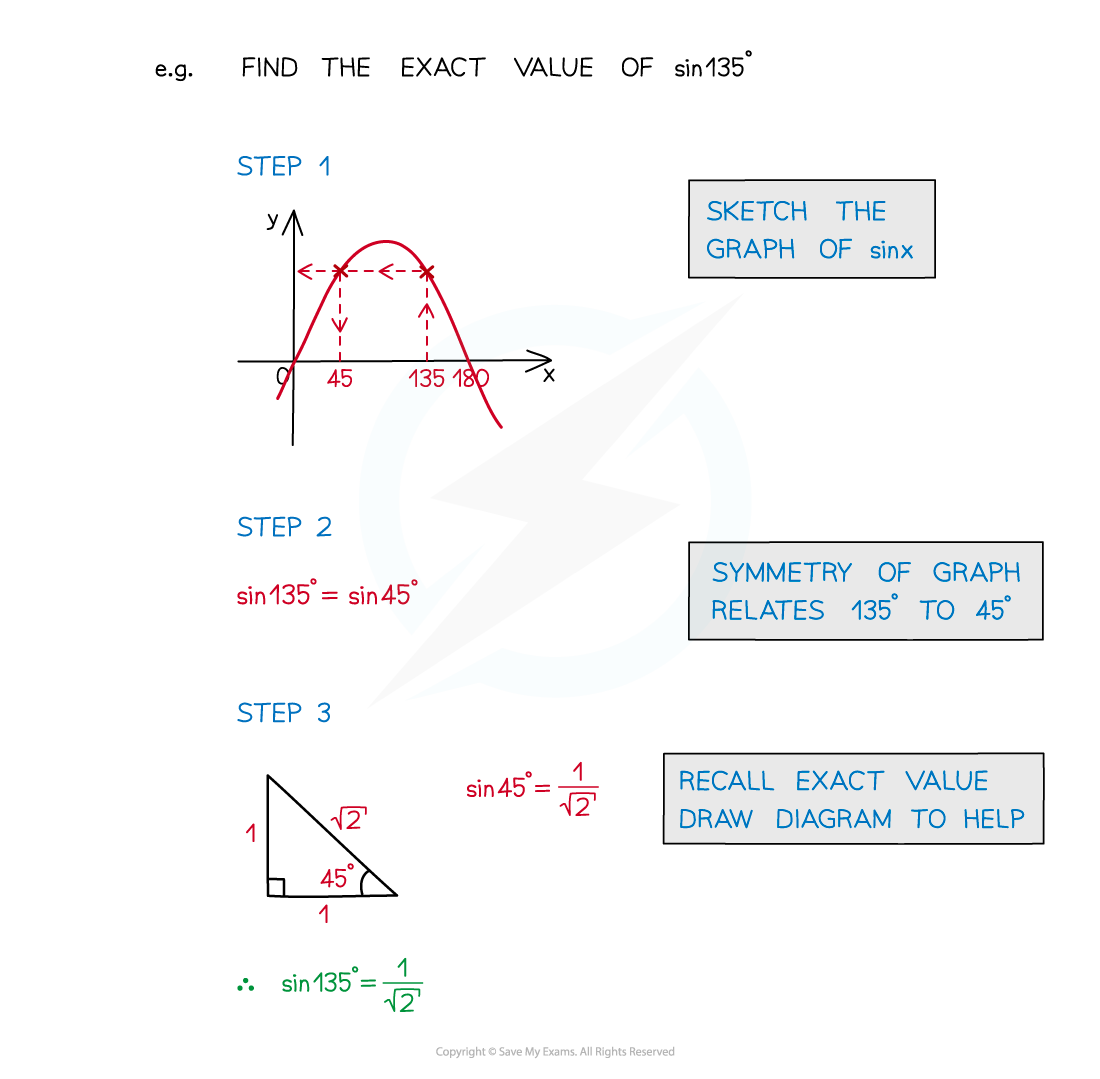#### Exam Tip

• Draw the triangles for sin, cos and tan of 30°, 45° and 60° on the exam paper so that you can then use them as many times as you need throughout the paper.

#### Worked Example